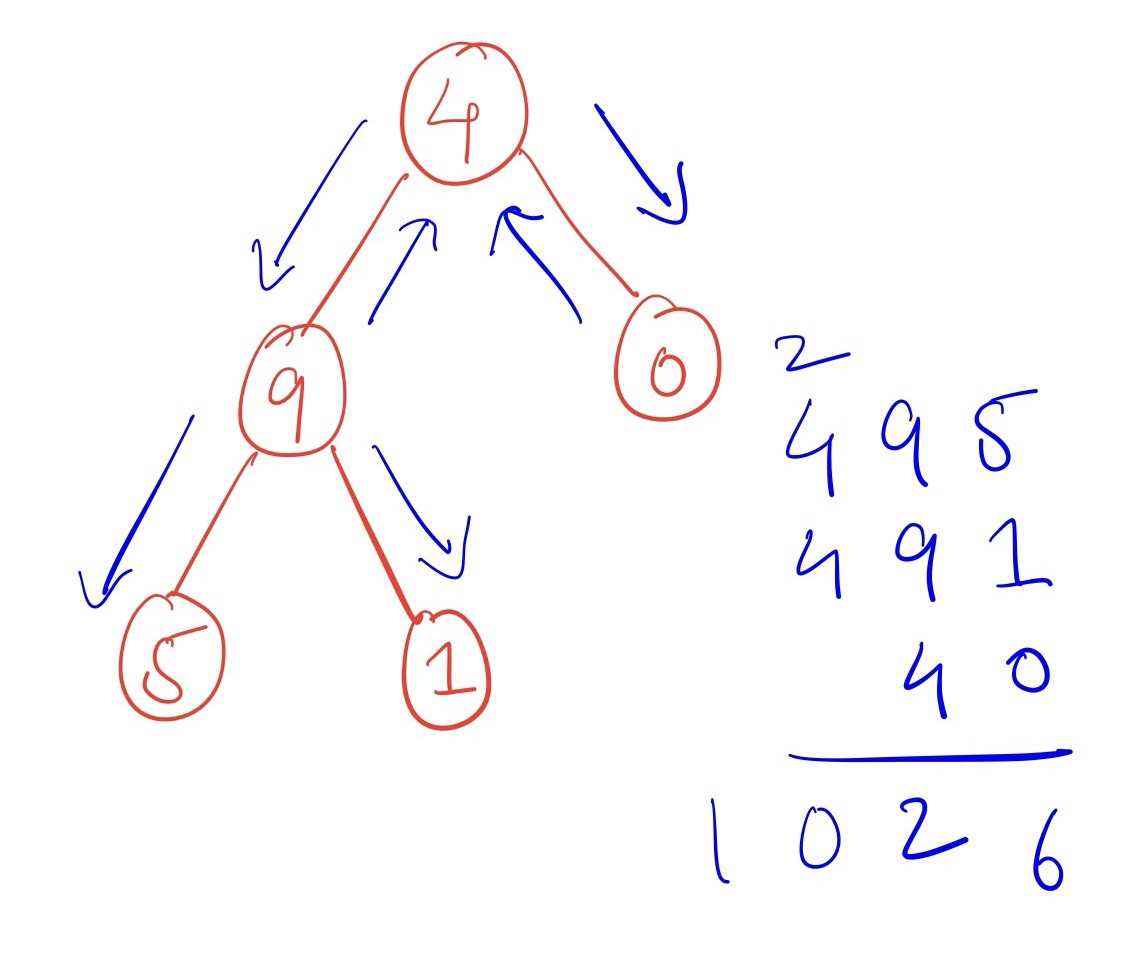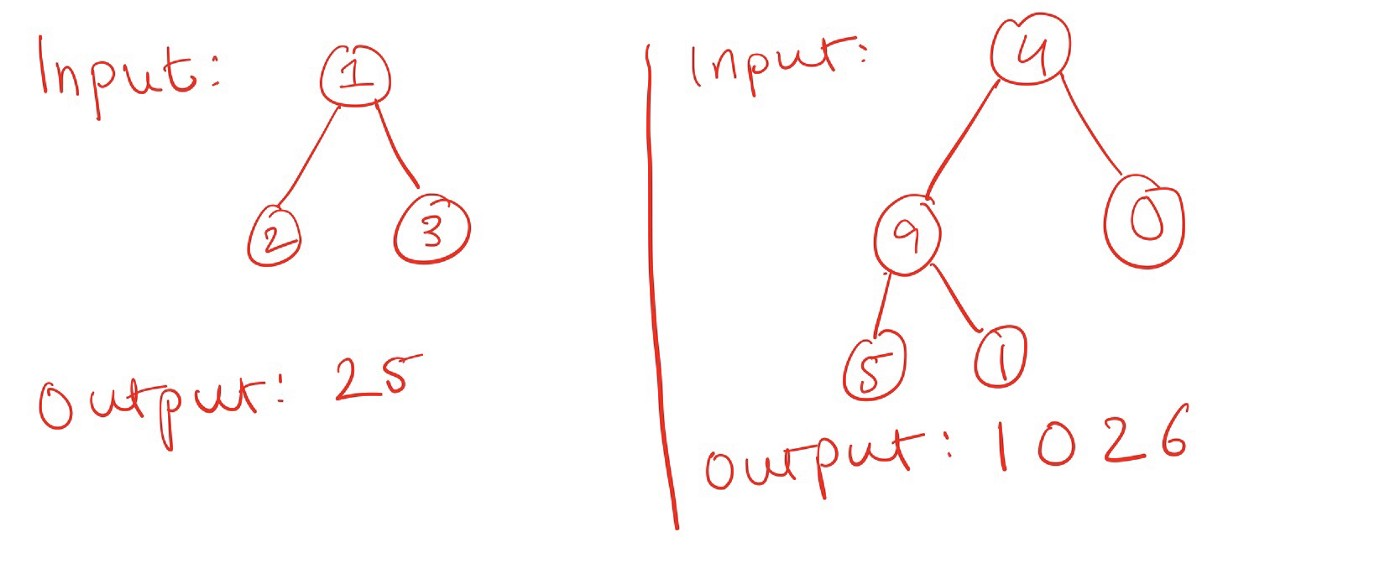# Summing Root To Leaf Numbers#### Raivat Shah

Student at NUS Computing

Sum Root to Leaf Numbers is an interesting problem from LeetCode. The problem is of medium difficulty and is about binary trees. This post is an explained solution to the problem.

I assume that you’re familiar with Python and the concept of binary trees. If you’re not, you can read this article to get started.## The Problem#

Given a binary tree whose nodes contain values `0-9`, we have to find the sum of all numbers formed by root-to-leaf paths. A leaf is a node that doesn’t have any child nodes. In a binary tree, a root-to-leaf path is always unique. Here below is the expected behavior of the solution required:In the tree on the left, the output is `25`. `25` is the sum of `12` and `13`, which are the two numbers formed when starting from `1` and visiting every leaf. In the tree on the right, the output is `1026` as it is the sum of the three numbers `495`, `491` and `40`.

## The Observations and Insights#

1. To construct a number, we traverse the tree from the root to the leaf, appending digits where the most significant digit is at the root, and the least significant digit is at the leaf. We visit some leaves before other nodes that are closer to the root. This suggests that a depth-first search will be useful.

2. The construction of numbers is incremental and similar of sorts: the only difference between `495` and `491` (from the tree on the right) is the last digit. If we remove the `5` and insert a `1` in its place, we have the next required number. A number essentially comprises of the leaf's digit appended to all the digits in ancestor nodes. Thus, numbers within the same subtree have common digits.

3. Finally, notice that this problem involves a tree, so a recursive solution is helpful.

## The Solution#

We can do a `pre-order` traversal of the tree where we incrementally construct a number and exploit the fact that numbers formed by nodes in the same sub-tree have common digits. When we’re done forming numbers in a sub-tree, we can backtrack and go to another sub-tree.

Let’s create a `Solution` class to encompass our solution.

class Solution:
def sum_numbers(self, root: TreeNode) -> int:

The method signature given to us in the problem has one argument: root, which is of the type `TreeNode` . A `TreeNode` class is as follows (from LeetCode):

class TreeNode:
def __init__(self, val=0, left=None, right=None):
self.val = val
self.left = left
self.right = right

From observation #2, notice that appending a node's digit to its ancestors can be achieved by moving all the digits of the number formed by ancestors to the right by 1 place and adding the current node's digit. The digits can be moved by multiplying the number formed by ancestors by 10 (since we're in base-10). For example:

`495 = 49 x 10 + 5`

Thus, we can keep track of the current digits in an integer. This is important because we won't incur extra storage space for higher input sizes. We can pass around this value in the function parameter itself. Since the method signature given can only have one parameter, let's create a `sum_root_to_leaf_helper` method.

We can think of the `sum_root_to_leaf_helper` method recursively and process each node differently based on whether or not it is a leaf.

• If the node is a leaf, we want to add its digit to our current digits by moving all the other digits to the right. We also want to return this value (since we'll backtrack from here).

• If it is not a leaf, we want to add the digit to our current digits by moving all the other digits to the right. We also want to continue constructing the number by traversing down this node's left and right subtrees.

If the current node is a `None`, we can simply return 0 because it doesn't count.

Thus, our `sum_root_to_leaf_helper` method will be as follows:

def sum_root_to_leaf_helper(node, partial_sum=0):
if not node:
return 0
partial_sum = partial_sum * 10 + node.val
# Leaf
if not node.left and not node.right:
return partial_sum
# Non Leaf
return (sum_root_to_leaf_helper(node.left, partial_sum) + \
sum_root_to_leaf_helper(node.right, partial_sum))

We use a default value for the partial sum to be 0.

In our main method, we want to include the `sum_root_to_leaf_helper` method as a nested method and simply pass on the node parameter. Finally, this is how our solution looks:

class Solution:
def sumNumbers(self, root: TreeNode) -> int:
def sum_root_to_leaf_helper(node, partial_sum=0):
if not node:
return 0
partial_sum = partial_sum * 10 + node.val
# Leaf
if not node.left and not node.right:
return partial_sum
# Non Leaf
return (sum_root_to_leaf_helper(node.left, partial_sum) + \
sum_root_to_leaf_helper(node.right, partial_sum))
return sum_root_to_leaf_helper(root)

## The Algorithmic Complexity#

When we come up with a solution, it is important to analyze its algorithmic complexity not only to estimate its performance but also to identify areas for improvement and reflect on our problem-solving skills. We should always ask the question: can we do better than X? Where X is the current complexity of our solution.

Time:

Our solution is a modification of the depth-first-search pre-order traversal where we visit all nodes exactly once and perform a trivial computation (moving digits by integer multiplication). Thus, our runtime is simply `O(N)` where `N` represents the number of nodes in the given tree. A solution better than `O(N)` doesn't seem possible because to construct a number from digits, we need to know all the digits (and thus visit all nodes).

Space:

In terms of storage, we incur a high cost in the recursion call stack that builds up as our `sum_root_to_leaf_helper` calls itself. These calls build-up as one waits for another to finish.

The maximum call stack is dependent upon the height of the binary tree (since we start backtracking after we visit a leaf), giving a complexity of `O(H)` where `H` is the height of the binary tree. In the worst case, the binary tree is skewed in either direction and thus `H = N`. Therefore, the worst-case space complexity is `O(N)`.

It is possible to do better than `O(N)` by using a Morris Preorder Traversal. The basic idea is to link a node and its predecessor temporarily. You can read more about it here.